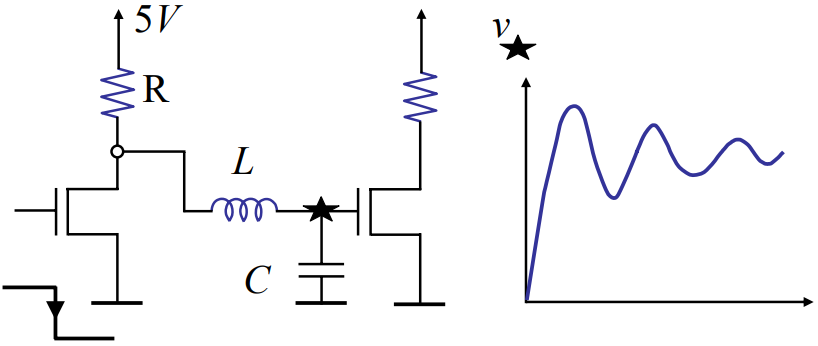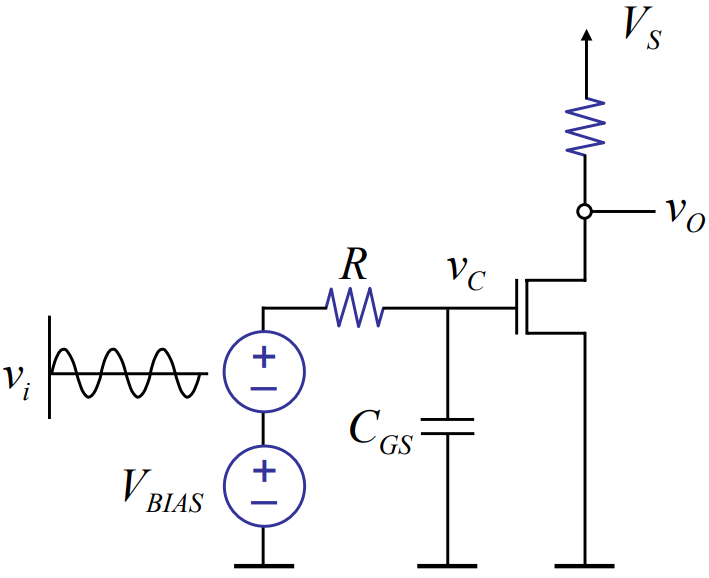## 正弦和稳态Today, look at response of networks to sinusoidal drive.

For motivation, consider our old friend, the amplifier：在偏置电压上加上一个小信号$$v_i$$来驱动，这是放大器电路的常用模板；电路中某戴维南电阻连接到信号源上。• Observe $$v_o$$ amplitude as the frequency of the input $$v_i$$ changes. Notice it decreases with frequency.
• Also observe $$v_o$$ shift as frequency changes (phase).
• 注意无论$$v_i$$怎么变化，$$v_c$$和$$v_o$$始终是反相的(180度相位差)